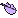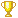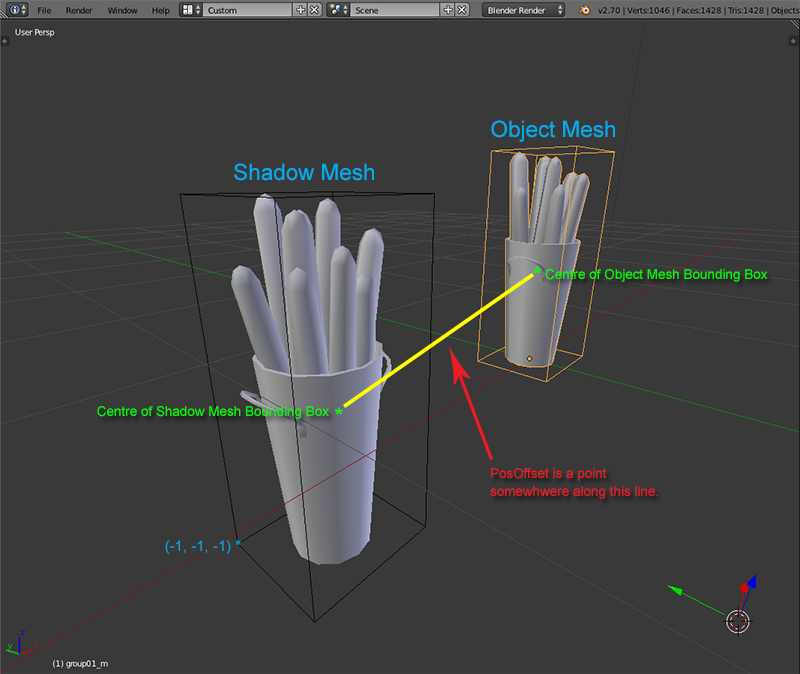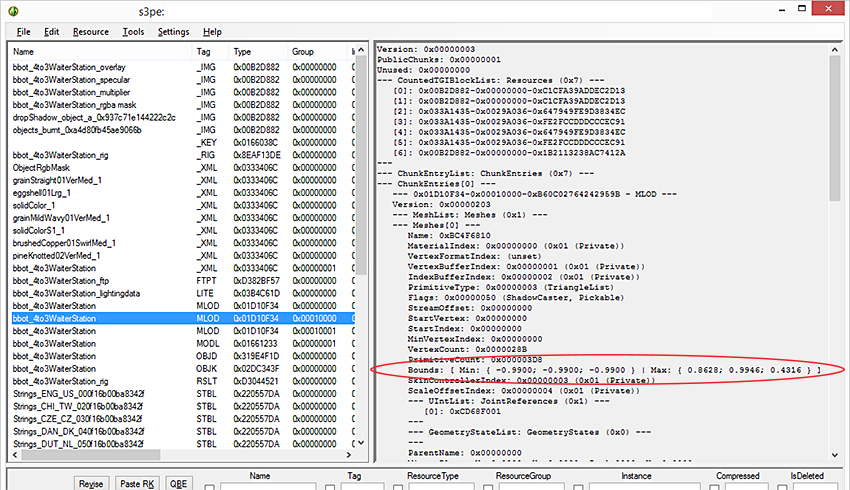Replies: 1 (Who?), Viewed: 7010 times.InstructorOriginal Poster
#115th Oct 2016 at 10:28 PM Last edited by Butterbot : 30th Dec 2017 at 9:36 PM. Reason: Correction of PosOffSet definition and step 2.3.5
[Tutorial] Creating Custom Sunshadows Without TSRW
I. Introduction

The following describes the creation of object sunshadows without the use of TSR Workshop.

TSRW has the ability to automatically generate shadow meshes and resulting sunshadows. However, it is unable to edit certain objects, such as items from the Store or some rabbitholes. Furthermore, as of this writing, the most recent, TS4-compatible versions of TSRW are reported to have ongoing issues with TS3 files, making its continued support of TS3 uncertain. For these reasons, it is useful to be able to obtain shadow meshes and correct sunshadows using alternate tools.

A note on terminology: in this tutorial, "shadow mesh" denotes the mesh derived from an in-game object. This mesh is used to project a flat shadow on the ground plane. "Sunshadow" refers to this 2D cast shadow.

II. Prerequisites

Working knowledge of:

III. Background + Overview

Objects that are large enough to cast shadows typically contain two meshes: one of the actual object, and a shadow mesh derived from the object mesh. The shadow mesh is created by resizing and displacing the object mesh. This transformation is described in detail by BloomsBase here and has been automated by cmomoney as part of Blender TS3 Tools' "Create Shadow Mesh" function. It is advisable to read through BloomsBase's thread for a better understanding of the process; at the very least, note that the shadow mesh is obtained by scaling up the object mesh by 200% on all axes and positioning the posterior inferior rightmost corner of its bounding box at (-1, -1, -1). A shadow mesh's maximum volume is 2x2x2 Blender Units (BU); if the scaled-up object mesh exceeds this volume, it must be resized on each axis to fit within these bounds.

The sunshadow is computed by the game using the shadow mesh and two additional parameters: PosOffset and PosScale (each is composed of values for x, y, and z). Adding a shadow mesh that has been correctly generated by Blender TS3 Tools to an object without also setting the appropriate values for these two parameters will result in improperly projected sunshadows.Figure 1: Both objects have the same shadow mesh, but the object at the bottom has an incorrectly projected sunshadow due to PosOffset/PosScale having the wrong values.

PosOffset can be conceptualized as a point somewhere along a line that connects the centre of the object mesh's bounding box to the centre of the shadow mesh's bounding box. The exact location of this point is affected by the scaling factor between the two meshes (each axis has its own factor, since large shadow meshes that are resized to fit into the 2x2x2 BU volume do not necessarily retain their proportions). Calculating PosOffset is thus, broadly speaking, a two-step process: 1) determine the centre point of the object bounding box and of the shadow mesh bounding box and 2) determine the exact coordinates of the point on the connecting line.Figure 2

For any one axis, given the size of the bounding boxes and the coordinate of their centres, the value for PosOffset can be obtained as follows, using the x-axis as example:

let
x = x coordinate of centre of object bounding box
x' = x coordinate of centre of shadow mesh bounding box
w = size of object bounding box on x-axis
w' = size of shadow mesh bounding box on x-axis

x coordinate of PosOffset = x - (w/w' * x')

PosScale has a default value of (1.525925E-05, 1.525925E-05, 1.525925E-05) if the shadow mesh (object mesh enlarged by 200%) fits within the 2x2x2 BU volume limit without being scaled down. If the shadow mesh has been downsized on any given axis to fit the 2x2x2 BU volume, the value of the PosScale on that axis is computed as follows, using the x-axis as example:

let
w = size of object bounding box on x-axis
w' = size of shadow mesh bounding box on x-axis

x value of PosScale = w'/w * 1.525925E-05

IV. Procedure

1. Generate the shadow mesh using Blender TS3 Tools.
1. Export the initial shadow mesh from S3PE as .s3m2b and import into Blender.
2. Import the object mesh into Blender.
3. Select the object mesh and click the "Make Shadow" button under Sims 3 MLOD/MODL Tools in the Scene panel.
4. Compare this newly generated shadow mesh with the original object (it might be useful to re-import the object mesh temporarily or to open it in another instance of Blender). If the shadow mesh looks somewhat crushed or squeezed, note on which axes that distortion has occurred, as these are the axes along which TS3 Tools had to scale down the shadow mesh to fit into a 2x2x2 BU volume (at this point, it's important to remember that the y and z axes are switched between Blender and TS3).
5. Verify that this newly generated shadow mesh really fits within 2x2x2 cube in Blender, as TS3 Tools for some reason adds a very small margin which can cause the mesh to overshoot this volume. If any part of the shadow mesh extends beyond this volume to any degree, move the affected vertices as minimally as possible back into the 2x2x2 BU bounds. Look out for this particularly in tall or wide objects. Failure to do so will lead to part of the sunshadow being cast in the wrong direction, e.g towards the light source or/and the centre of the mesh.Figure 3: Example of Blender-generated shadow mesh needing manual downscaling

6. Select both the initial shadow mesh and the newly generated shadow mesh. Click the "Swap" button under Sims 3 MLOD/MODL Tools in the Scene panel.
7. Delete the original shadow mesh.
8. Export this new shadow mesh from Blender and import it back into S3PE. Click OK when prompted to update the bounding box.
2. Compute the new values for PosOffset.
1. Obtain object mesh bounding box values. This can be done in two ways:
• In Blender, select the object mesh, navigate to the Object panel, and make sure that "Bounds" under Display is checked (the adjacent dropdown should show "Box"). In the 3D viewport, place the 3D cursor on any corner of the now visible bounding box, zooming in to ensure best accuracy, and note its xyz coordinates. Move the 3D cursor to the opposing diagonal corner of the bounding box and note the coordinates. Because the y and z axes are switched between Blender and TS3, exchange the positions of the y and z coordinates.Figure 4: Example of corner pair from which to obtain bounding box coordinates

• In S3PE, select the object mesh MLOD or MODL, and click the "Grid" button. Expand ChunkEntries ->  ChunkEntries -> RCOLBlock -> Meshes to display the mesh groups contained in the resource. Expand the mesh entry that contains the object mesh (usually,  Meshes is the dropshadow mesh, with the actual object mesh located after; check the PrimitiveCount line for a hint, as the dropshadow mesh typically has a very low polycount.) If the object mesh is composed of several mesh groups, inspect the one that is largest, most encompassing in volume, as that is likely the main determinant of the size of the bounding box. Expand Bounds, and note the xyz coordinates in both Min and Max entries.
2. Obtain shadow mesh bounding box values by selecting the shadow mesh MLOD in S3PE. In the preview pane on the right, locate the line labeled "Bounds" and note the associated coordinates.Figure 5

3. Compute the value of PosOffset for the x-axis.
1. Determine the x coordinate of centre of object bounding box (x).
1. Take the x values of the bounding box coordinates obtained from the object mesh. Let the larger value be Max and the smaller value be Min.
2. x = (Max - Min) / 2 + Min
2. Determine the x coordinate of centre of shadow mesh bounding box (x').
1. Take the x values of the bounding box coordinates obtained from the shadow mesh. Let the larger value be Max' and the smaller value be Min'.
2. x' = (Max' - Min') / 2 + Min'
3. Determine the size of object bounding box on x-axis (w).
1. Take the x values of the bounding box coordinates obtained from the object mesh. Let the larger value be Max and the smaller value be Min.
2. w = Max - Min
4. Determine the size of shadow mesh bounding box on x-axis (w').
1. Take the x values of the bounding box coordinates obtained from the shadow mesh. Let the larger value be Max' and the smaller value be Min'.
2. w' = Max' - Min'
5. Value of PosOffset for the x-axis = x - (w/w' * x')
4. Compute the values of PosOffset for the y and z axes by adapting the steps above.
3. Compute the new values for PosScale. There are two scenarios:
• If the Blender-generated shadow mesh did not need to be downscaled to fit into a 2x2x2 BU volume, use the default values of (1.525925E-05, 1.525925E-05, 1.525925E-05).
• If the Blender-generated shadow mesh needed to be downscaled, whether manually or by TS3 Tools, for each axis on which this was performed, calculate the PosScale value as follows: w'/w * 1.525925E-05. For any axis on which scaling down was unnecessary, the default value of 1.525925E-05 should be used. Remember that the y and z axes are switched between Blender and TS3, so, for example, if the shadow mesh were downscaled on the z-axis in Blender, it's the y coordinate of PosScale that needs to be calculated.
4. Set the new values for PosOffset and PosScale.
1. In S3PE, select the shadow mesh MLOD and locate the "PosOffset" and "PosScale" entries in the preview pane on the right. Note the chunk entry number and field entry numbers.Figure 5

2. Click the "Grid" button. Expand ChunkEntries -> chunk entry noted previously -> RCOLBlock -> Mtnf -> SData -> field entries noted previously.
3. Enter the newly calculated PosOffset and PosScale values (Data0 is x, Data1 is y, Data2 is z; leave Data3 unchanged).
4. Commit the changes and save the .package file.

Many thanks to @BloomsBase for sharing his findings and to @cmomoney for incorporating them into Blender TS3 Tools.

A selection of threads with relevant discussions:

http://modthesims.info/t/388978#216th Oct 2016 at 9:27 AM